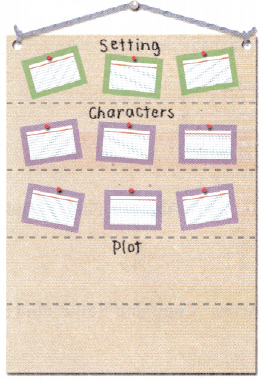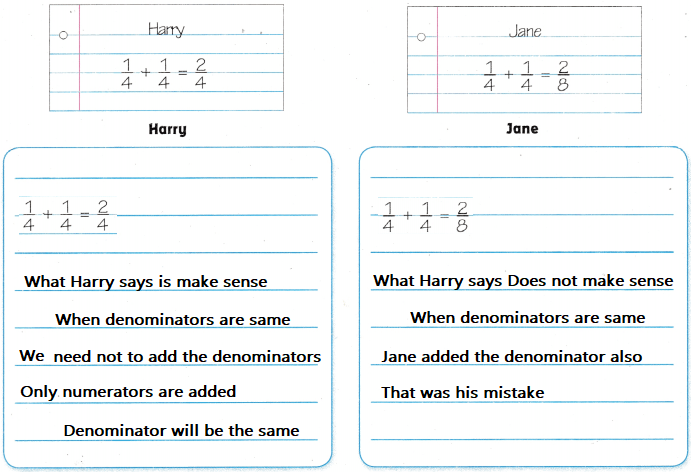Refer to our Texas Go Math Grade 4 Answer Key Pdf to score good marks in the exams. Test yourself by practicing the problems from Texas Go Math Grade 4 Lesson 5.5 Answer Key Add and Subtract Fractions.

Essential Question

How can you add and subtract fractions with like denominators?
Explanation:
If the denominators are same we can directly add or subtract the denominators

Unlock the Problem

Julie is making a poster for a book report. The directions say to use $$\frac{1}{5}$$ of the poster to describe the setting, $$\frac{2}{5}$$ of the poster to describe the characters, and the rest of the poster to describe the plot. What part of the poster will she the to describe the plot?$$\frac{1}{5}$$ + $$\frac{2}{5}$$  = $$\frac{3}{5}$$
1 – $$\frac{3}{5}$$  = $$\frac{2}{5}$$

Example Use a model.

Shade _______ to represent the part for the setting.
Shade _______ to represent the part for the characters.Shade $$\frac{1}{5}$$ to represent the part for the setting.
Shade $$\frac{2}{5}$$  to represent the part for the characters.
• Write an equation for the part of the poster used for the setting and characters. ____________
$$\frac{1}{5}$$ + $$\frac{2}{5}$$  = $$\frac{3}{5}$$

• What does the part of the model that is not shaded represent? ____________
$$\frac{2}{5}$$ the part of the model that is not shaded represent

• Write an equation for the part of the poster she will use for the plot. ____________
1 – $$\frac{3}{5}$$  = $$\frac{2}{5}$$
equation for the part of the poster she will use for the plot.

• So, Julie will use ____________ of the poster to describe the plot.
$$\frac{2}{5}$$
Julie will use $$\frac{2}{5}$$ of the poster to describe the plot.

Math Talk

Mathematical Processes
Why should Julie divide her poster into 5 equal parts instead of 3 equal parts? Explain.
So, According to the content
she divide her poster into 5 equal parts instead of 3 equal parts

Question 1.
What’s the Error? Luke says $$\frac{1}{5}+\frac{2}{5}=\frac{3}{10}$$. Describe his error.
Explanation:
$$\frac{1}{5}+\frac{2}{5}=\frac{3}{10}$$.
In this equation the denominators are same
so, we will not add the denominators

Share and Show

Question 1.
9 twelfth-size parts – 5 twelfth-size parts = 4 twelfth-size parts
$$\frac{9}{12}-\frac{5}{12}$$ = ____________
$$\frac{9}{12}-\frac{5}{12}$$ = $$\frac{4}{12}$$
The difference of $$\frac{9}{12}-\frac{5}{12}$$ is $$\frac{4}{12}$$

Question 2.
$$\frac{3}{12}+\frac{8}{12}$$ = __________
$$\frac{3}{12}+\frac{8}{12}$$ = $$\frac{11}{12}$$
Explanation:
It is reasonable because $$\frac{11}{12}$$ is greater than 0 and lesser than 1

Question 3.
$$\frac{1}{3}+\frac{1}{3}$$ = __________
$$\frac{1}{3}+\frac{1}{3}$$ = $$\frac{2}{3}$$
Explanation:
It is reasonable because $$\frac{2}{3}$$ is greater than 0 and lesser than 1

Question 4.
$$\frac{3}{4}-\frac{1}{4}$$ = __________
$$\frac{3}{4}-\frac{1}{4}$$ = $$\frac{2}{4}$$
Explanation:
It is reasonable because $$\frac{2}{4}$$ is greater than 0 and lesser than 1

Question 5.
$$\frac{2}{6}+\frac{2}{6}$$ = __________
$$\frac{2}{6}+\frac{2}{6}$$ = $$\frac{4}{6}$$
Explanation:
It is reasonable because $$\frac{4}{6}$$ is greater than 0 and lesser than 1

Question 6.
$$\frac{3}{8}+\frac{1}{8}$$ = __________
$$\frac{3}{8}+\frac{1}{8}$$ = $$\frac{4}{8}$$
Explanation:
It is reasonable because $$\frac{4}{8}$$ is greater than 0 and lesser than 1

Question 7.
$$\frac{6}{10}-\frac{2}{10}$$ = __________
$$\frac{6}{10}-\frac{2}{10}$$ = $$\frac{4}{10}$$
Explanation:
It is reasonable because $$\frac{4}{10}$$ is greater than 0 and lesser than 1

Problem Solving

H.O.T. Algebra Find the unknown fraction.

Question 8.
$$\frac{2}{8}$$ + _________ = $$\frac{6}{8}$$
$$\frac{6}{8}$$ – $$\frac{2}{8}$$  = $$\frac{4}{8}$$
Explanation:
Found the unknown fraction.

Question 9.
1 – ____________ = $$\frac{3}{4}$$
1 – $$\frac{1}{4}$$= $$\frac{3}{4}$$
Explanation:
Found the unknown fraction.

Question 10.
$$\frac{4}{5}$$ – ___________ = $$\frac{1}{5}$$
$$\frac{4}{5}$$–$$\frac{1}{5}$$ = $$\frac{3}{5}$$
$$\frac{4}{5}$$ – $$\frac{3}{5}$$= $$\frac{1}{5}$$
Explanation:
Found the unknown fraction.

Practice: Copy and Solve Find the sum or difference.

Question 11.
$$\frac{1}{4}+\frac{1}{4}$$ = __________
$$\frac{1}{4}+\frac{1}{4}$$ = $$\frac{2}{4}$$
Explanation:
Found the sum of the fraction.

Question 12.
$$\frac{9}{10}-\frac{5}{10}$$ = __________
$$\frac{9}{10}-\frac{5}{10}$$ = $$\frac{4}{10}$$
Explanation:
Found the difference of the fraction.

Question 13.
$$\frac{1}{12}+\frac{7}{12}$$ = __________
$$\frac{1}{12}+\frac{7}{12}$$ = $$\frac{8}{12}$$
Explanation:
Found the sum of the fraction.

Question 14.
Use Math Language Multi-Step A city worker is painting a stripe clown the center of Main Street. Main Street $$\frac{8}{10}$$ is mile long. The worker painted $$\frac{4}{10}$$ mile of the street. Explain how to find what part of a mile is left to paint.
$$\frac{8}{10}$$ – $$\frac{4}{10}$$  = $$\frac{4}{10}$$
Explanation:
$$\frac{4}{10}$$ part of a mile is left to paint

Question 15.
The length of a rope was $$\frac{6}{8}$$ yard. Jeff cut the rope into 3 pieces. Each piece is a different length measured in eighths of a yard. What is the length of each piece of rope?
$$\frac{2}{8}$$ + $$\frac{2}{8}$$  + $$\frac{2}{8}$$  = $$\frac{6}{8}$$
$$\frac{2}{8}$$  is the length of each piece of rope

Problem Solving

Question 16.
H.O.T. Sense or Nonsense? Brian says that when you add or subtract fractions with the same denominator, you can add or subtract the numerators and keep the same denominator. Is Brian correct? Explain.
Explanation:
It make sense what Brian says

H.O.T. Multi-Step Sense or Nonsense?

Question 17.
Harry says that $$\frac{1}{4}+\frac{1}{4}=\frac{2}{4}$$. Jane says $$\frac{1}{4}+\frac{1}{4}=\frac{2}{8}$$. Whose answer makes sense? Whose answer is nonsense? Explain your reasoning.Question 18.
The Small family has 1 pie to share for dessert. The parents eat $$\frac{1}{6}$$ of the pie. The children eat $$\frac{5}{6}$$ of the pie. What part of the pie does the family eat?
(A) $$\frac{6}{6}$$
(B) $$\frac{5}{12}$$
(C) $$\frac{5}{6}$$
(D) $$\frac{3}{12}$$
Explanation:
$$\frac{1}{6}$$ + $$\frac{5}{6}$$ = $$\frac{6}{6}$$

Question 19.
Ana rides her bike $$\frac{5}{10}$$ mile to the park. Then she rides $$\frac{3}{10}$$ mile to the soccer field. How far does Ana ride her bike?
(A) $$\frac{2}{10}$$ mile
(B) $$\frac{2}{20}$$ mile
(C) $$\frac{8}{10}$$ mile
(D) $$\frac{8}{20}$$ mile
Explanation:
$$\frac{5}{10}$$ + $$\frac{3}{10}$$  = $$\frac{8}{10}$$ mile
$$\frac{8}{10}$$ mile far  Ana ride her bike

Question 20.
Multi-Step Christopher mixes $$\frac{3}{8}$$ gallon of red paint with $$\frac{5}{8}$$ gallon of blue paint to make purple paint. He uses $$\frac{2}{8}$$ gallon of purple paint to paint bookshelves. How much purple paint is left?
(A) $$\frac{8}{8}$$ gallon
(B) $$\frac{4}{8}$$ gallon
(C) $$\frac{10}{8}$$ gallon
(D) $$\frac{6}{8}$$ gallon
Explanation:
$$\frac{3}{8}$$ + $$\frac{5}{8}$$ = $$\frac{8}{8}$$ gallon
$$\frac{8}{8}$$ gallon – $$\frac{2}{8}$$ gallon = $$\frac{6}{8}$$ gallon
$$\frac{6}{8}$$ gallon purple paint is left

TEXAS Test Prep

Question 21.
Otis has 1 cup of granola. He added $$\frac{3}{8}$$ cup to a bowl of yogurt.
How much granola is left?
(A) $$\frac{3}{8}$$ cup
(B) $$\frac{2}{8}$$ cup
(C) $$\frac{5}{8}$$ cup
(D) $$\frac{8}{8}$$ cup
Explanation:
1 – $$\frac{3}{8}$$ = $$\frac{5}{8}$$ cup granola is left

### Texas Go Math Grade 4 Lesson 5.5 Homework and Practice Answer Key

Question 1.
$$\frac{2}{7}+\frac{3}{7}$$ = ____________
$$\frac{2}{7}+\frac{3}{7}$$ = $$\frac{5}{7}$$
Explanation:
It is reasonable because $$\frac{5}{7}$$ is greater than 0 and lesser than 1

Question 2.
$$\frac{10}{12}-\frac{6}{12}$$ = ____________
$$\frac{10}{12}-\frac{6}{12}$$ = $$\frac{4}{12}$$
Explanation:
It is reasonable because $$\frac{4}{12}$$  is greater than 0 and lesser than 1

Question 3.
$$\frac{8}{9}-\frac{5}{9}$$ = ____________
$$\frac{8}{9}-\frac{5}{9}$$ = $$\frac{3}{9}$$
Explanation:
It is reasonable because $$\frac{3}{9}$$ is greater than 0 and lesser than 1

Question 4.
$$\frac{4}{8}+\frac{4}{8}=$$ = ____________
$$\frac{4}{8}+\frac{4}{8}=$$ = 1
Explanation:
It is reasonable because the sum is 1 is greater than 0 and equal to 1

Find the sum or difference.

Question 5.
$$\frac{4}{7}-\frac{1}{7}$$ = ____________
$$\frac{4}{7}-\frac{1}{7}$$ = $$\frac{3}{7}$$
Explanation:
The difference of two fractions is found

Question 6.
$$\frac{1}{6}+\frac{5}{6}$$ = ____________
$$\frac{1}{6}+\frac{5}{6}$$ = $$\frac{6}{6}$$ = 1
Explanation:
The sum of two fractions is found

Find the unknown fraction.

Question 7.
$$\frac{1}{5}$$ + ________ = $$\frac{4}{5}$$
$$\frac{4}{5}$$ – $$\frac{1}{5}$$ = $$\frac{3}{5}$$
Explanation:
Found the unknown fraction.

Question 8.
1 – ________ = $$\frac{2}{4}$$
$$\frac{2}{4}$$
Explanation:
1 – $$\frac{2}{4}$$ = $$\frac{2}{4}$$
Found the unknown fraction.

Question 9.
$$\frac{3}{8}$$ + ________ = 1
$$\frac{3}{8}$$ + $$\frac{1}{8}$$ = 1
Explanation:
Found the unknown fraction.

Question 10.
$$\frac{5}{6}$$ – ________ = $$\frac{2}{6}$$
$$\frac{5}{6}$$ – $$\frac{2}{6}$$ = $$\frac{3}{6}$$
Explanation:
Found the unknown fraction.

Problem Solving

Question 11.
Jorge’s rain gauge showed that it rained $$\frac{3}{8}$$inch during the first week of the month. At the end of the second week the gauge was $$\frac{7}{8}$$ inch full. How much did it rain during the second week?
$$\frac{7}{8}$$ – $$\frac{3}{8}$$ = $$\frac{4}{8}$$
Explanation:
$$\frac{4}{8}$$ it rain during the second week

Question 12.
Courtney watched a caterpillar crawl on the sidewalk. It crawled $$\frac{4}{10}$$ yard and stopped. The caterpillar crawled $$\frac{5}{10}$$ yard further. How far did the caterpillar crawl in all?
$$\frac{4}{10}$$ + $$\frac{5}{10}$$ = $$\frac{9}{10}$$

Lesson Check

Question 13.
At Mary’s party, $$\frac{4}{8}$$ of the guests had chocolate ice cream and $$\frac{3}{8}$$ of the guests had strawberry ice cream. What fraction of the ice cream was either chocolate or strawberry?
(A) $$\frac{1}{16}$$
(B) $$\frac{7}{8}$$
(C) $$\frac{1}{8}$$
(D) $$\frac{7}{16}$$
Explanation:
$$\frac{4}{8}$$ –  $$\frac{3}{8}$$  = $$\frac{1}{8}$$
Explanation:
$$\frac{1}{8}$$ fraction of the ice cream was either chocolate or strawberry

Question 14.
The fuel gauge in Mrs. Jensen’s car showed $$\frac{3}{4}$$ of a tank of gas. After driving into the city and buck, the gauge showed $$\frac{1}{4}$$ of a tank of gas. how much gas did Mrs. Jensen use?
(A) $$\frac{2}{4}$$ tank
(B) 1 tank
(C) $$\frac{1}{3}$$ tank
(D) $$\frac{3}{4}$$ tank
Explanation:
$$\frac{3}{4}$$ – $$\frac{1}{4}$$ = $$\frac{2}{4}$$ tank
$$\frac{2}{4}$$ tank gas that Mrs. Jensen use

Question 15.
Craig is folding laundry for his family. In the basket, $$\frac{1}{6}$$ of the shirts belong to his older brother and $$\frac{3}{6}$$ of the shirts belong to his younger brother. What fraction of the shirts belong to either his older or younger brother?
(A) $$\frac{6}{6}$$
(B) $$\frac{5}{6}$$
(C) $$\frac{2}{3}$$
(D) $$\frac{1}{3}$$
Explanation:
$$\frac{1}{6}$$ + $$\frac{3}{6}$$ = $$\frac{4}{6}$$
1 – $$\frac{4}{6}$$ = $$\frac{2}{6}$$
$$\frac{1}{3}$$ fraction of the shirts belong to either his older or younger brother

Question 16.
After sharing with the class, Dana has $$\frac{4}{12}$$ of her cupcakes left. What fractional part of the cupcakes did she share with the class?
(A) $$\frac{2}{3}$$
(B) $$\frac{1}{4}$$
(C) $$\frac{3}{4}$$
(D) $$\frac{1}{3}$$
Explanation:
1 – $$\frac{4}{12}$$  = $$\frac{8}{12}$$ = $$\frac{1}{3}$$
$$\frac{1}{3}$$ fractional part of the cupcakes did she share with the class

Question 17.
Multi-Step Josie had 1 gallon of ice cream. She used $$\frac{3}{10}$$ gallon to make a chocolate milkshake and $$\frac{3}{10}$$ gallon to make a vanilla milkshake. How much ice cream is left?
(A) $$\frac{6}{10}$$ gallon
(B) $$\frac{4}{10}$$ ga11on
(C) $$\frac{7}{10}$$ gallon
(D) $$\frac{5}{10}$$ galIon
Explanation:
$$\frac{3}{10}$$ + $$\frac{3}{10}$$ = $$\frac{6}{10}$$
1 – $$\frac{6}{10}$$ = $$\frac{4}{10}$$ gallon ice cream is left

Question 18.
Multi-Step In Mr. Green’s music class, $$\frac{3}{12}$$ of the students play the guitar and $$\frac{4}{12}$$ of the students play the violin. The rest of the students play the piano. What fraction of the students play the piano?
(A) $$\frac{8}{12}$$
(B) $$\frac{9}{12}$$
(C) $$\frac{7}{12}$$
(D) $$\frac{5}{12}$$
$$\frac{3}{12}$$ + $$\frac{4}{12}$$  = $$\frac{7}{12}$$
1 – $$\frac{7}{12}$$ = $$\frac{5}{12}$$
$$\frac{5}{12}$$ fraction of the students play the piano